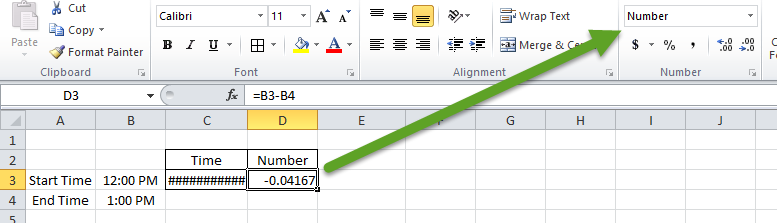# Calculating Hours Difference of Time in Excel SpreadsheetsWhen working with Time in Microsoft Excel you may have noticed that there is a problem when you subtract certain numbers.  Excel gives you an error, but it doesn’t look like an error.  Excel just shows you a lot of hastags (Pound symbols) like this: “#############”.  And they are never ending.  Most errors display #N/A, #ERROR or #VALUE.  So what is going on here?

## Negative Time

Now you may or may not know, but time moves on and not back (at least not until they develop that time machine).  However, definitely in Excel terms, time is only positive.  So if you subtract 2 values of time and they are negative, you will see the error like this: “#############”.

Here is a sample when we subtract a later time like 1PM from Noon as you see below:

A B C
1 Time
2 Start Time 12:00 PM ###########
3 End Time 1:00 PM
Worksheet Formulas

Cell Formula
C2 =B2-B3

## Techniques for Dealing with Negative Time

There is a simple tip and trick to get around the strange time error that you are seeing when you subtract time in Excel.  All you have to do is to change the Number Format of the cell where you are subtracting time.Yes, that is it.  Notice that I have the same formulas below in cells C2 and D2 however, one cell is showing an error and the other is showing a number.  The only difference is the number format that you can change from the Excel Home Ribbon.

A B C D
1 Time Number
2 Start Time 12:00 PM ########### -0.04167
3 End Time 1:00 PM
Worksheet Formulas
Cell Formula
C2 =B2-B3
D2 =B2-B3

## Determining Time Worked by Subtracting a Lunch Hour

So lets take it one step further.  If you need to subtract time to calculate something like the total time worked today minus the non-paid lunch hour, then use this technique.

If we subtract time as per the previous answer, we will not get the answer we desire as Excel treats an hour as a decimal.  It works really well, but your answer would be “0.04167” and not 1.

To get around this, you may want to use the HOUR Function.  This hour subtraction formula would wrap both cell references in HOUR Function look like this:

A B C
1 Hour Function
2 Start Time 12:00 PM -1.00000
3 End Time 1:00 PM
Worksheet Formulas
Cell Formula
C2 =HOUR(B2)-HOUR(B3)

## Video Demonstration

Check out the Video Tutorial here:

Hopefully this helped you think of time in Excel in a different way and help you with your time issues.  If so, please leave me a comment below.

Steve=True

1.Bengt-Göran

If it is important that it looks like a timestamp you could use a formula like this for presentation (but not for further calculations):

=IF(D7<D8,"-","")&TEXT(ABS(D7-D8),"[h]:mm:ss")

•SteveEqualsTrue

Thanks for the comment. Good technique. Thanks!

2.ravi

great one steve as always. KUDOS

Text(b2-a2,”-h:mm:ss”)

B2= start date
a2 = end date

or wrap this in IF formula

•SteveEqualsTrue

Thanks Ravi

3. # If you found the website and tutorials helpful, please consider donating to keep the lights on.

## Donate with PayPal here:4.Mike Malley

I am trying to figure out an excel formula to calculate our military time difference keeping it to whole half hours.
Example:
start time end time hours total hours
0800 1200 4.0 4.0
1230 1700 4.5 8.5
0800 1200 4.0 12.5
1230 1700 4.5 25.0

Is this even possible?

•SteveEqualsTrue

Hi Mike. Yes, this should be possible. Note that 1= 0.0416667 in an Excel date/time value. YOu can find this if you enter a time like 12:00 AM in 1 cell and 1:00 AM in another cell. Then subtract the 2 values and change the format of that cell to a regular number vs a time format. You will see this value for 1 hour. 0.0416667 1/2 hour would be 1/2 of that value. So you can add and subtract values as you see fit. One issue you may run into is times that span midnight, so you will have to work out that as an issue possibly with ABS() Function.